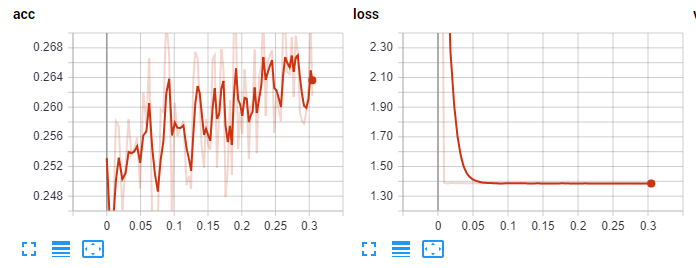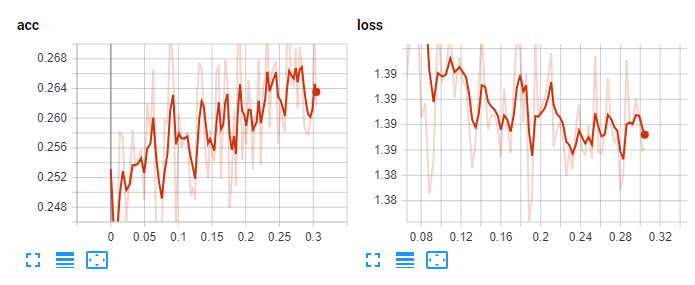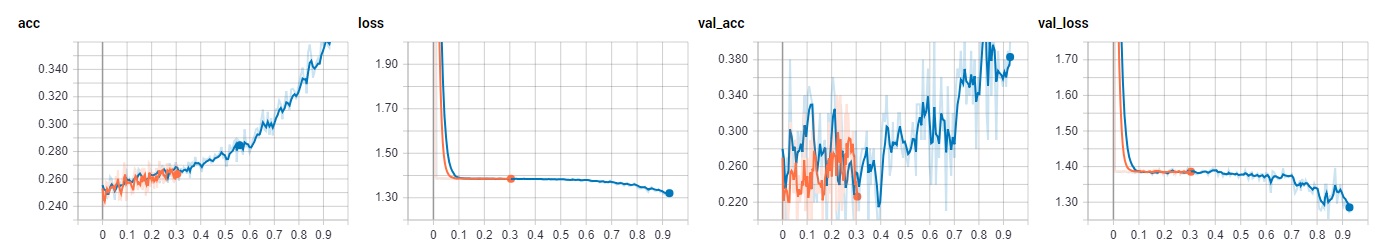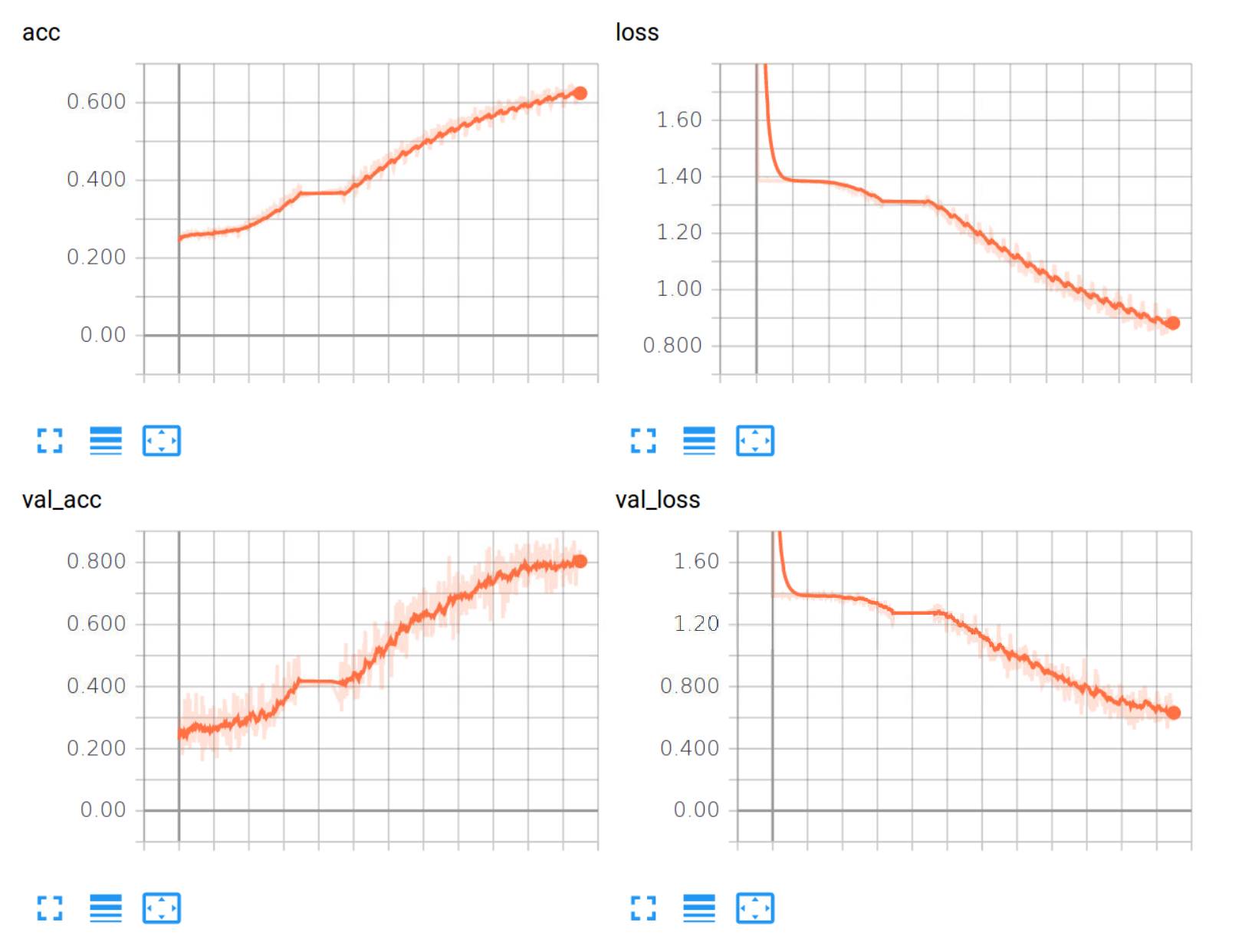## Training Neural Network Model - Python AI in StarCraft II p.11

Welcome to Part 11 of the creating and Artificial Intelligence bot in StarCraft II with Python series. In this tutorial, we're going to be working on actually feeding our training data through our network. Because our training data is sufficiently large, we need to take extra measures to do this smoothly.

To start, while Keras has the ability for us to pass epochs, Keras simply wont be aware of our full dataset in order to do this. There's probably some nice way to batch load, I just don't know it, so I am just going to do it myself.

So, to begin, we're going to specify our total desired epochs. I will go with 10 for now. We can always circle back and continue building on the same model. If, after 10 epochs, we're not happy, we can always load the 10 epoch model and add 3, 10, or 300 more epochs to it easily.

```hm_epochs = 10

for i in range(hm_epochs):```

In this case, we're going to begin iterating over some block of code 10 times. This block of code is going to iterate through all of our training data, loading in the individual files, and training. Each individual file is a StarCraft II game where the actions and map were logged if the random AI (randomly choosing our 4 attack options) defeated the Hard AI.

Next, we want to start going through all of our training data, but we need to do it in chunks in order to not have memory issues.

```for i in range(hm_epochs):
current = 0
increment = 200
not_maximum = True```

The way we will go in chunks is to take a poll of all of the files (os.listdir), and then begin slicing our way through that entire list in increments of 200 files (200 games at a time).

```    all_files = os.listdir(train_data_dir)
maximum = len(all_files)
random.shuffle(all_files)```

We need to know the maximum, so that we don't iterate beyond the max. There's definitely a better way to code this logic, but this is what I've got for now.

With our 200 games at a time, we're ready to build and balance that section of training data to feed to the network:

```    while not_maximum:
print("WORKING ON {}:{}".format(current, current+increment))
no_attacks = []
attack_closest_to_nexus = []
attack_enemy_structures = []
attack_enemy_start = []

for file in all_files[current:current+increment]:
full_path = os.path.join(train_data_dir, file)
data = list(data)
for d in data:
choice = np.argmax(d)
if choice == 0:
no_attacks.append([d, d])
elif choice == 1:
attack_closest_to_nexus.append([d, d])
elif choice == 2:
attack_enemy_structures.append([d, d])
elif choice == 3:
attack_enemy_start.append([d, d])```

We want to balance this data next, so each choice has as many occurences as the other so there aren't any bias issues within the network. So we want a function that will determine the lengths for us. I want to just do something like:

`        lengths = check_data()`

So now we're going to build that `check_data` function:

```def check_data():
choices = {"no_attacks": no_attacks,
"attack_closest_to_nexus": attack_closest_to_nexus,
"attack_enemy_structures": attack_enemy_structures,
"attack_enemy_start": attack_enemy_start}

total_data = 0

lengths = []
for choice in choices:
print("Length of {} is: {}".format(choice, len(choices[choice])))
total_data += len(choices[choice])
lengths.append(len(choices[choice]))

print("Total data length now is:",total_data)
return lengths```

Again, not really the most ideal way to do this (referencing lists instead of passing them). Once we have the lengths returned, we want to grab the lowest length, and, after shuffling all of our attack choices training datas, we want to set them all to that length:

```        lowest_data = min(lengths)

random.shuffle(no_attacks)
random.shuffle(attack_closest_to_nexus)
random.shuffle(attack_enemy_structures)
random.shuffle(attack_enemy_start)

no_attacks = no_attacks[:lowest_data]
attack_closest_to_nexus = attack_closest_to_nexus[:lowest_data]
attack_enemy_structures = attack_enemy_structures[:lowest_data]
attack_enemy_start = attack_enemy_start[:lowest_data]

check_data()```

Since we jumped around a bit, here's the full script up to this point so far, just to make sure you're following right:

```import keras  # Keras 2.1.2 and TF-GPU 1.8.0
from keras.models import Sequential
from keras.layers import Dense, Dropout, Flatten
from keras.layers import Conv2D, MaxPooling2D
from keras.callbacks import TensorBoard
import numpy as np
import os
import random

model = Sequential()

input_shape=(176, 200, 3),
activation='relu'))

activation='relu'))

activation='relu'))

learning_rate = 0.0001

model.compile(loss='categorical_crossentropy',
optimizer=opt,
metrics=['accuracy'])

tensorboard = TensorBoard(log_dir="logs/STAGE1")

train_data_dir = "train_data"

def check_data():
choices = {"no_attacks": no_attacks,
"attack_closest_to_nexus": attack_closest_to_nexus,
"attack_enemy_structures": attack_enemy_structures,
"attack_enemy_start": attack_enemy_start}

total_data = 0

lengths = []
for choice in choices:
print("Length of {} is: {}".format(choice, len(choices[choice])))
total_data += len(choices[choice])
lengths.append(len(choices[choice]))

print("Total data length now is:",total_data)
return lengths

# if you want to load in a previously trained model
# that you want to further train:
hm_epochs = 10

for i in range(hm_epochs):
current = 0
increment = 200
not_maximum = True
all_files = os.listdir(train_data_dir)
maximum = len(all_files)
random.shuffle(all_files)

while not_maximum:
print("WORKING ON {}:{}".format(current, current+increment))
no_attacks = []
attack_closest_to_nexus = []
attack_enemy_structures = []
attack_enemy_start = []

for file in all_files[current:current+increment]:
full_path = os.path.join(train_data_dir, file)
data = list(data)
for d in data:
choice = np.argmax(d)
if choice == 0:
no_attacks.append([d, d])
elif choice == 1:
attack_closest_to_nexus.append([d, d])
elif choice == 2:
attack_enemy_structures.append([d, d])
elif choice == 3:
attack_enemy_start.append([d, d])

lengths = check_data()
lowest_data = min(lengths)

random.shuffle(no_attacks)
random.shuffle(attack_closest_to_nexus)
random.shuffle(attack_enemy_structures)
random.shuffle(attack_enemy_start)

no_attacks = no_attacks[:lowest_data]
attack_closest_to_nexus = attack_closest_to_nexus[:lowest_data]
attack_enemy_structures = attack_enemy_structures[:lowest_data]
attack_enemy_start = attack_enemy_start[:lowest_data]

check_data()```

Continuing along now, we now want to add all of these choices together in one larger list:

```        train_data = no_attacks + attack_closest_to_nexus + attack_enemy_structures + attack_enemy_start
```

Now we want to shuffle this list again, or the network will likely just learn to predict the last thing over and over:

```        random.shuffle(train_data)
print(len(train_data))```

Now we've got the data, let's feed it in!

```        test_size = 100
batch_size = 128```

We will reserve 100 samples for validation (though these will not be out of sample, due to the shuffle. Eventually, you'd want to setup some truly out of sample data), and the network will train in batches of 128. Let's setup and shape our data:

```        x_train = np.array([i for i in train_data[:-test_size]]).reshape(-1, 176, 200, 3)
y_train = np.array([i for i in train_data[:-test_size]])

x_test = np.array([i for i in train_data[-test_size:]]).reshape(-1, 176, 200, 3)
y_test = np.array([i for i in train_data[-test_size:]])```

Fit the data:

```        model.fit(x_train, y_train,
batch_size=batch_size,
validation_data=(x_test, y_test),
shuffle=True,
verbose=1, callbacks=[tensorboard])```

Save the model and continue iteration:

```        model.save("BasicCNN-{}-epochs-{}-LR-STAGE1".format(hm_epochs, learning_rate))
current += increment
if current > maximum:
not_maximum = False```

Running this, I began with testing on ~6,000 samples, which was only about 200 games, just to see, and there was definitely no learning to be had. I increased to 110K samples, and things began to improve.

Initial tensorboard stats:Zoomed in:From here, I tried more layers, less layers, more lr, less lr, different optimizers, different activations, nothing better than the above. Okay, let's run 10 epochs then and see if we continue to learn.

After settling with 200 files at a time, batches in 128, 1e-4 learning rate and 10 epochs:Great! At this point, I spun up a Volta 100 on PaperSpace to do some quicker testing.

The model I ended with here can be downloaded: Stage 1 neural network

The training stats:Looking good enough. Let's see how this does in the next tutorial.

Full code up to this point:

```import keras  # Keras 2.1.2 and TF-GPU 1.9.0
from keras.models import Sequential
from keras.layers import Dense, Dropout, Flatten
from keras.layers import Conv2D, MaxPooling2D
from keras.callbacks import TensorBoard
import numpy as np
import os
import random

model = Sequential()

input_shape=(176, 200, 3),
activation='relu'))

activation='relu'))

activation='relu'))

learning_rate = 0.0001

model.compile(loss='categorical_crossentropy',
optimizer=opt,
metrics=['accuracy'])

tensorboard = TensorBoard(log_dir="logs/STAGE1")

train_data_dir = "train_data"

def check_data():
choices = {"no_attacks": no_attacks,
"attack_closest_to_nexus": attack_closest_to_nexus,
"attack_enemy_structures": attack_enemy_structures,
"attack_enemy_start": attack_enemy_start}

total_data = 0

lengths = []
for choice in choices:
print("Length of {} is: {}".format(choice, len(choices[choice])))
total_data += len(choices[choice])
lengths.append(len(choices[choice]))

print("Total data length now is:", total_data)
return lengths

hm_epochs = 10

for i in range(hm_epochs):
current = 0
increment = 200
not_maximum = True
all_files = os.listdir(train_data_dir)
maximum = len(all_files)
random.shuffle(all_files)

while not_maximum:
print("WORKING ON {}:{}".format(current, current+increment))
no_attacks = []
attack_closest_to_nexus = []
attack_enemy_structures = []
attack_enemy_start = []

for file in all_files[current:current+increment]:
full_path = os.path.join(train_data_dir, file)
data = list(data)
for d in data:
choice = np.argmax(d)
if choice == 0:
no_attacks.append([d, d])
elif choice == 1:
attack_closest_to_nexus.append([d, d])
elif choice == 2:
attack_enemy_structures.append([d, d])
elif choice == 3:
attack_enemy_start.append([d, d])

lengths = check_data()
lowest_data = min(lengths)

random.shuffle(no_attacks)
random.shuffle(attack_closest_to_nexus)
random.shuffle(attack_enemy_structures)
random.shuffle(attack_enemy_start)

no_attacks = no_attacks[:lowest_data]
attack_closest_to_nexus = attack_closest_to_nexus[:lowest_data]
attack_enemy_structures = attack_enemy_structures[:lowest_data]
attack_enemy_start = attack_enemy_start[:lowest_data]

check_data()

train_data = no_attacks + attack_closest_to_nexus + attack_enemy_structures + attack_enemy_start

random.shuffle(train_data)
print(len(train_data))

test_size = 100
batch_size = 128

x_train = np.array([i for i in train_data[:-test_size]]).reshape(-1, 176, 200, 3)
y_train = np.array([i for i in train_data[:-test_size]])

x_test = np.array([i for i in train_data[-test_size:]]).reshape(-1, 176, 200, 3)
y_test = np.array([i for i in train_data[-test_size:]])

model.fit(x_train, y_train,
batch_size=batch_size,
validation_data=(x_test, y_test),
shuffle=True,
verbose=1, callbacks=[tensorboard])

model.save("BasicCNN-{}-epochs-{}-LR-STAGE1".format(hm_epochs, learning_rate))
current += increment
if current > maximum:
not_maximum = False
```

The next tutorial:• Introduction and Collecting Minerals - Python AI in StarCraft II p.1

• Workers and Pylons - Python AI in StarCraft II p.2

• Geysers and Expanding - Python AI in StarCraft II p.3

• Building an AI Army - Python AI in StarCraft II p.4

• Commanding your AI Army - Python AI in StarCraft II p.5

• Defeating Hard AI - Python AI in StarCraft II p.6

• Deep Learning with SC2 Intro - Python AI in StarCraft II p.7

• Scouting and more Visual inputs - Python AI in StarCraft II p.8

• Building our training data - Python AI in StarCraft II p.9

• Building Neural Network Model - Python AI in StarCraft II p.10

• Training Neural Network Model - Python AI in StarCraft II p.11
• Using Neural Network Model - Python AI in StarCraft II p.12

• Version 2 Changes - Python AI in StarCraft II p.13

• Improving Scouting - Python AI in StarCraft II p.14

• Adding Choices - Python AI in StarCraft II p.15

• Visualization Changes - Python AI in StarCraft II p.16

• More Training and Findings - Python AI in StarCraft II p.17